# Transforms¶

## Overview¶

Signal Transformations allow for taking signals and further processing the data such as running math on, complex functions, and combining with other signals. The output is always one or more unique signals for the asset that can be used like any other signal.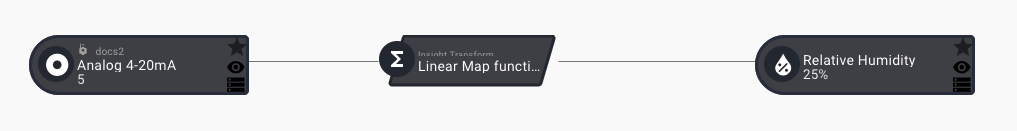Transformations convert a signal to a new signal with some math applied, a join with other signals, or with more complex processing. The output of a transformation is a unique signal that can be used as a part of the asset for rules, dashboards, etc.

Off the Shelf transforms available within ExoSense

Function Type Description
Linear Gain Standard Linear gain + offset function.
Linear Map Standard Map to new value based on a linear scale.
Join Standard Join multiple signals with basic operations.
Continuous Accumulation Standard Accumulate/Decrement signal values
Infinite Impulse Response (IIR) Standard Infinite Impulse Response
Negative Temperature Coefficient (NTC) Standard Calculate Temperature from NTC sensor
Band Gap Filter Standard Filter in / out of a band gap
Total Mass from Flow Mass Flow Get Total Mass from Flow Mass over time
Total Volume from Flow Volume per Minute Flow Get Volume from Flow Volume over time
Total Volume from Flow Volume per Second Flow Get Volume from Flow Volume over time
Aggregation by Time Interval Windowed Aggregate values by time interval
Rolling Aggregation by Count Windowed Rolling aggregation by count
Rolling Aggregation by Duration Windowed Rolling aggregation by a time duration

## Using Transforms¶

In the Modify Asset Config interface for any given Asset, transforms can be added to the existing signals. Once added, one or more new signals are created.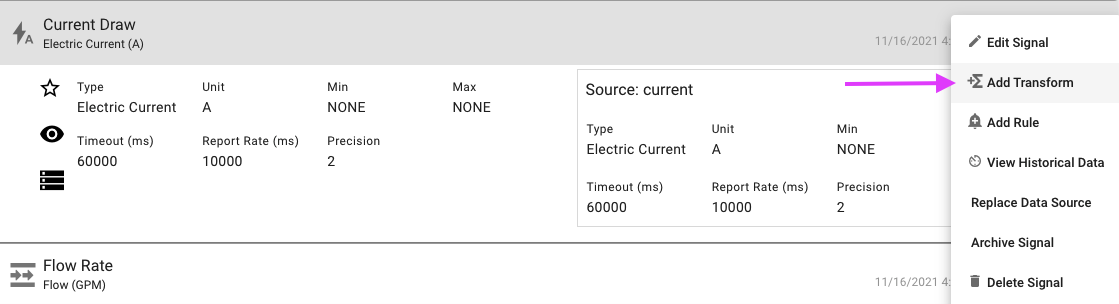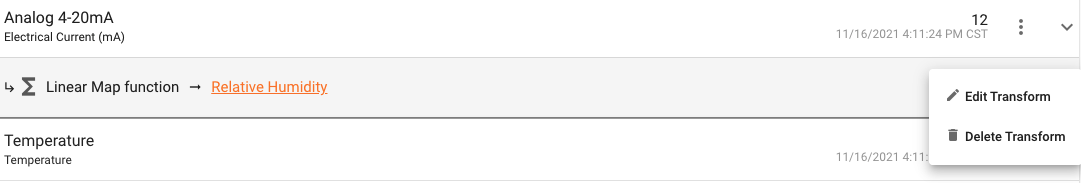The output Signals will be listed in Modify Asset Configuration under 'Calculated Signals' and are available for adding rules to, further transformations or analytics, reporting, and dashboard use.

The Exosite IoT Marketplace contains additional Insight modules that can be added to ExoSense by administrators. Custom transformations can be built for transforming of data or analytics. Once added or created, these show up in the Modify Asset Config 'Add Transform' interface.

Custom Transforms

The off-the-shelf standard transformations provided with ExoSense may not cover all use cases. Custom transforms can be created using the ExoSense Insight functionality. You can build your own transforms using the Insight capabilities.

## Standard Transform Details¶

### Linear Gain¶

Allows for applying a scaling factor and offset for a signal using the function: `result = gain * input + offset`.

Use Cases:

• Use Linear Scale for applying calibration factors to signals.

Configuration

I/O Details
Inputs 1 Numeric signal
Outputs 1 Numeric signal (Type / Unit can be configured)

Parameters:

Parameter Format Required Description
Gain Number ✔︎ Multiplier of the signal value before the offset is applied. Default to 1. Can be negative.
Offset Number ✔︎ Added to the value after the gain is applied. Default to 0. Can be negative. Can be negative.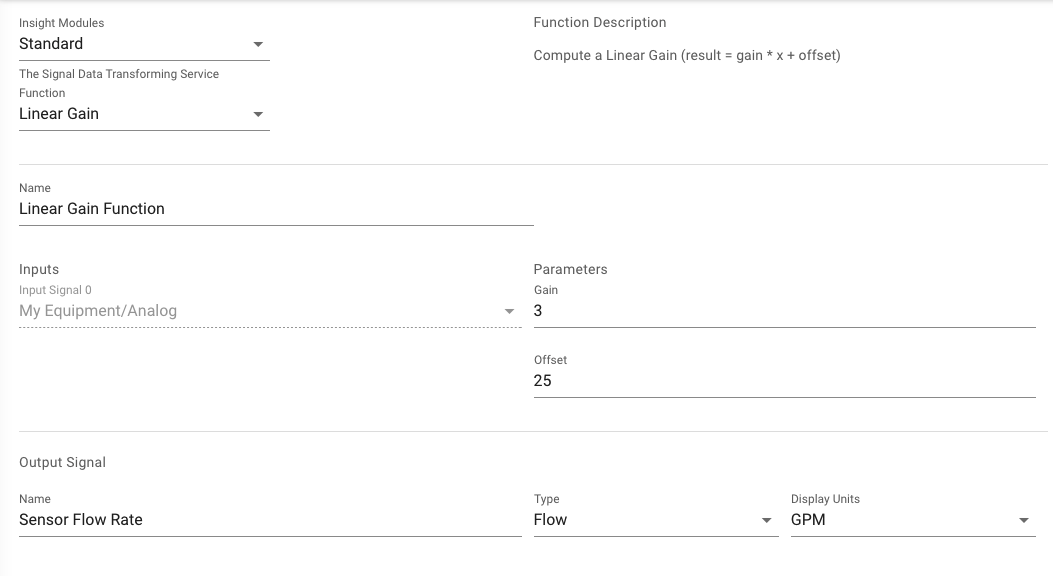### Linear Map¶

Map a signal to new values, based on a linear scale. Enter the original signal's minimum and maximum, and the transformed signal's minimum and maximum. Computes the Linear Map from `In Minimum` and `In Maximum` to `Out Minimum` and `Out Maximum` (result = (x - inMin) * ∆Out / ∆In + outMin)

Use Cases:

• Use Linear Mapping to transform a hardwired analog input 4-20mA signal to a 0-100% range signal.

Configuration

I/O Details
Inputs 1 Numeric signal
Outputs 1 Numeric signal (Type / Unit can be configured)

Parameters:

Parameter Format Required Description
In Minimum Number ✔︎ Minimum input value to use in the mapping function.
In Maximum Number ✔︎ Maximum input value to use in the mapping function.
Out Minimum Number ✔︎ Minimum output value to use in the mapping function.
Out Maximum Number ✔︎ Maximum outputs value to use in the mapping function.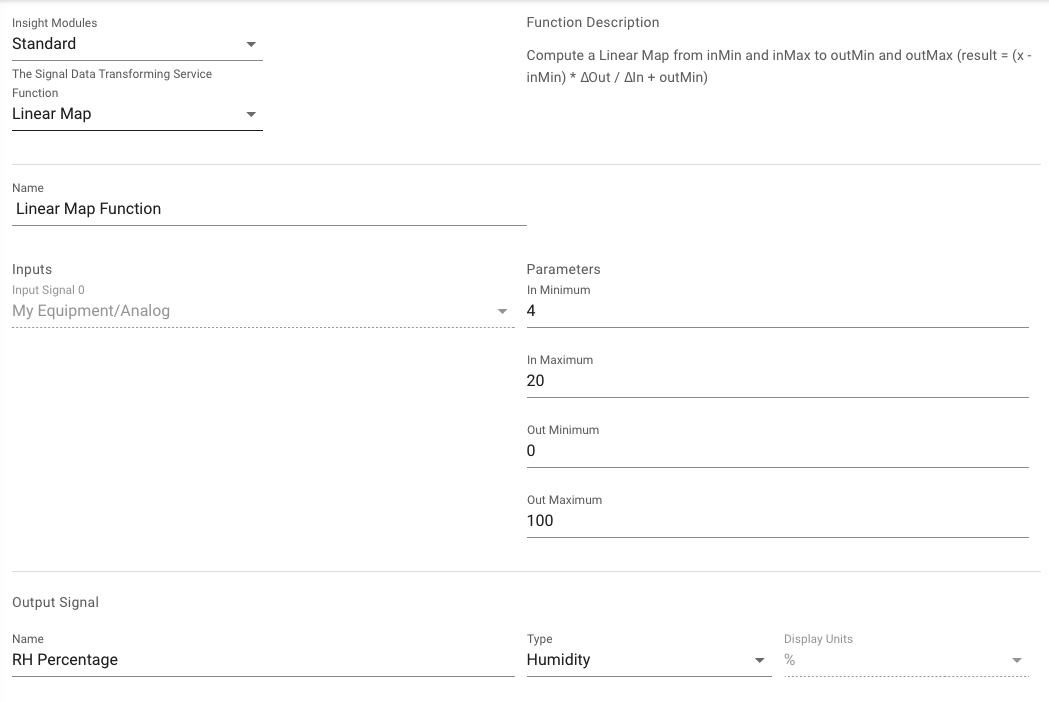### Join¶

Join multiple signals with basic math operations.

• subtraction
• multiply
• divide
• modulo

Evaluation Sequence

Join operations are evaluated top-to-bottom, rather than per standard order of operations.

Triggers

The join transform is triggered for each input signal value, meaning that if both signals have data written to them through a device at the same time, the transform will run twice. If your Join has two signals, A and B, and both values are written into the system at the same time, the function will for example run once for input A (using a previous B value) and once for input B (with the latest A value).

Use Cases:

• Example: Use Join to add energy meters together for a combined energy usage signal.

Configuration

I/O Details
Inputs 2 to 5 Numeric signals
Outputs 1 Numeric signal (Type / Unit can be configured)

Parameters:

Parameter Format Required Description
First Op Number ✔︎ Math operations to be applied between the First input and Second input.
Second Op Number Math operations to be applied between the Second input and Third input.
Third Op Number Math operations to be applied between the Third input and Fourth input.
Fourth Op Number Math operations to be applied between the Fourth input and Fifth input.
Gain Number ✔︎ Default is 1. Multiplier of the signal value before the offset is applied. Default to 1. Can be negative.
Offset Number ✔︎ Default is 0. Added to the value after the gain is applied. Default to 0. Can be negative. Can be negative.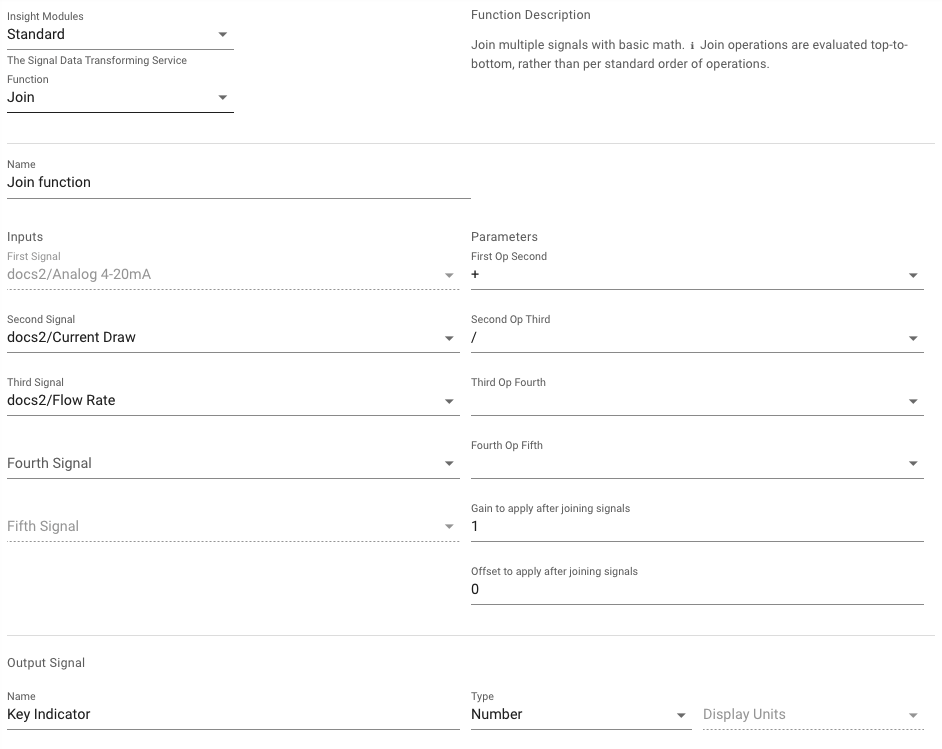### Continuous Accumulation¶

This function will Sum (Increment) or Subtract (Decrement) incoming values with an optional starting value (default 0).

Use Cases:

• Continuous accumulation of a sensor value over the lifetime

Configuration

I/O Details
Inputs 1 Numeric signal
Outputs 1 Numeric signal (Type / Unit can be configured)

Parameters:

Parameter Format Required Description
Value to start from Number ✔︎ Default 0. The starting number for increment/decrementing from

### Infinite Impulse Response (IIR)¶

This function will combine incoming values by an IIR [https://en.wikipedia.org/wiki/Infinite_impulse_response].

Configuration

I/O Details
Inputs 1 Numeric signal
Outputs 1 Numeric signal (Type / Unit can be configured)

Parameters:

Parameter Format Required Description
Weighting Value Number ✔︎ Decimal value between 0 and 1. A value between 0 and 1 excluding to weight prior values

### Negative Temperature Coefficient (NTC)¶

Computes Temperature from an analog voltage value using NTC provided Beta and Resistance at 25°C parameters. [https://en.wikipedia.org/wiki/Temperature_coefficient]

Configuration

I/O Details
Inputs 1 Numeric signal (assumes an analog voltage)
Outputs 1 Temperature signal (℃)

Parameters:

Parameter Format Required Description
Beta Number ✔︎ Beta value from sensor datasheet
Resistance at 25℃ Number ✔︎ Resistance at 25℃ value from sensor datasheet

### Band Gap Filter¶

This function will sort values that are inside and outside of a range into two output signals.

Configuration

I/O Details
Inputs 1 Numeric signal
Outputs 2 signals using the original input signal type/unit

Parameters:

Parameter Format Required Description
Lower Limit Number ✔︎ Exclusive lower limit for band gap
Upper Limit Number ✔︎ Exclusive upper limit for band gap

## Flow Transformations:¶

### Total Mass from Flow Mass¶

Derives the total mass from incoming flow mass rate.

Configuration

I/O Details
Inputs 1 signal of Flow Mass type
Outputs 1 signal of Mass type

### Total Volume from Flow Volume per Minute¶

Derives the total volume from incoming flow volume per minute.

Configuration

I/O Details
Inputs 1 signal of Flow Volume type, units of per minute
Outputs 1 signal of Volume type

### Total Volume from Flow Volume per Second¶

Derives the total volume from incoming flow volume per second.

Configuration

I/O Details
Inputs 1 signal of Flow Volume type, units of per second
Outputs 1 signal of Volume type

## Windowed Transforms:¶

### Aggregation by Time Interval¶

Aggregates signal values over the time window specified.

Configuration

I/O Details
Inputs 1 Numeric signal
Outputs 1 Numeric signal (Type / Unit can be configured)

Parameters:

Parameter Format Required Description
Interval Size Number ✔︎ Interval in seconds for the time window for aggregation
Interval Alignment Number ✔︎ Alignment in Seconds since Unix Epoch to align intervals against
How to combine the values Enum operations ✔︎ Operation for aggregating the data (min, max, mean, sum, count )

### Rolling Aggregation by Count¶

Rolling aggregation of past signal values by count.

Configuration

I/O Details
Inputs 1 Numeric signal
Outputs 1 Numeric signal (Type / Unit can be configured)

Parameters:

Parameter Format Required Description
Number of past values to combine Number ✔︎ Number of values to continuously combine with each new value as a rolling aggregation
How to combine the values Enum operations ✔︎ Operation for aggregating the data (min, max, mean, sum )

### Rolling Aggregation by Duration¶

Rolling aggregation of past signal values in the past time duration (in seconds).

Configuration

I/O Details
Inputs 1 Numeric signal
Outputs 1 Numeric signal (Type / Unit can be configured)

Parameters:

Parameter Format Required Description
Duration in seconds Number ✔︎ Time duration to continuously combine as a rolling aggregation
How to combine the values Enum operations ✔︎ Operation for aggregating the data (min, max, mean, sum )